# Abstract

In this study, mixed finite element models of plate bending are developed to include other variables (e.g., the membrane forces and shear forces) in addition to the generalized displacements to investigate their effect on nonlinear response. Various finite element models are developed using the weighted-residual statements of suitable equations. The classical plate theory and the first-order shear deformation plate theory are used in this study and the von Karman nonlinear strains are accounted for. Each newly developed model is examined and compared with displacement finite element models to evaluate their performance. Numerical results show that the new mixed models developed herein show better accuracy than existing displacement based models.

plates; nonlinear analysis; finite elements; mixed nodes; least-squares method

Novel mixed finite element models for nonlinear analysis of plates

Wooram Kim+ * Author email: jnreddy@tamu.edu + currently at Department of Mechanical Engineering, Korea Army Academy at Yeong cheon, Yeong cheon, 770-849 South Korea ; J. N. Reddy* * Author email: jnreddy@tamu.edu + currently at Department of Mechanical Engineering, Korea Army Academy at Yeong cheon, Yeong cheon, 770-849 South Korea

Advanced Computational Mechanics Laboratory, Department of Mechanical Engineering, Texas A&M University, College Station, TX 77843-3123 - USA

ABSTRACT

In this study, mixed finite element models of plate bending are developed to include other variables (e.g., the membrane forces and shear forces) in addition to the generalized displacements to investigate their effect on nonlinear response. Various finite element models are developed using the weighted-residual statements of suitable equations. The classical plate theory and the first-order shear deformation plate theory are used in this study and the von Karman nonlinear strains are accounted for. Each newly developed model is examined and compared with displacement finite element models to evaluate their performance. Numerical results show that the new mixed models developed herein show better accuracy than existing displacement based models.

Keywords: plates, nonlinear analysis, finite elements, mixed nodes, least-squares method.

1 INTRODUCTION

The basic idea of mixed finite element model is to treat stresses or stress resultants as dependent unknowns in addition to the generalized displacements. Certain mixed finite element models of plates were developed more than two decades ago by Putcha and Reddy [3, 4] to overcome the drawbacks of the displacement based models. The mixed finite element models [3, 4] were developed in the past by including bending moments as independent variables to reduce the differentiability of the transverse displacement. The mixed models can provide the same level of accuracy for the bending moments as that for the displacements, whereas in the displacement based model the bending moments are calculated at points other than nodes in the postprocessing. Thus, the displacement finite element models cannot provide the same level of accuracy for force-like variables as the mixed finite element models.

The objective of this study is to investigate the performance of finite element models based on weighted-residual formulations of the equations governing classical and first-order shear deformation plate theories. In particular, the study investigates merits and demerits of the newly developed mixed finite element models. The von Karman nonlinear equations [6, 9, 10] are used to develop alternative finite element models to the conventional displacement-based finite element models [5, 7, 8].

In the present study, mixed finite element models are developed to include other variables (i.e., the membrane forces and shear forces) in addition to the bending moments, and to see the effect of them on the nonlinear analysis. The effect of including other variables will be compared with different mixed models to show the advantage of the one type of model over other models. Two different mixed models based on the classical plate theory and two mixed models based on the first-order shear deformation plate theory are developed. The performance of the newly developed finite element models is evaluated by comparing the solutions with those of the existing displacement finite element models [9, 10].

2 REVIEW OF PLATE THEORIES

Here we derive governing equations of the classical plate theory (CPT) and first-order shear deformation theory (FSDT) of plates with the von Karman strains. The principle of virtual displacements is used to derive the equilibrium equations in terms of the stress resultants and then the stress resultants are expressed in terms of the displacements using elastic constitutive relations. We only summarize the pertinent equations in this section without presenting the details of the derivation.

The classical plate theory (CPT) is based on the Kirchhoff hypothesis, which consists of the following three assumptions: (1) straight lines perpendicular to the mid-surface (i.e. transverse normals) before deformation, remain straight after deformation; (2) the transverse normals do not experience elongation (i.e. they are in-extensible); (3) the transverse normals rotate such that they remain perpendicular to the mid-surface after deformation. On the other hand, the first-order shear deformation plate theory (FSDT) is based on the assumption the normals before deformation do not remain normal after deformation. Thus, the major difference between the kinematics of the CPT and FSDT is that the normality condition of CPT is relaxed in the FSDT, as illustrated in Fig. 1.

The equations of equilibrium expressed in terms of the stress resultants are the same in both theories, and they are given bywhere the stress resultants are defined byHere h denotes the total thickness of the plate and the (x,y)-plane is taken to coincide with the middle plane of the plate and the z-coordinate is taken perpendicular to the plane of the plate. The difference in the kinematics of each plate theory is responsible for the difference in the relationships between the stress resultants and the generalized displacements.

2.1 The Classical Plate Theory

The displacement field of the CPT is given by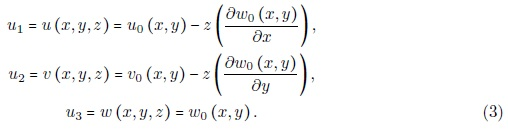Under the assumption of small strain but moderately large rotation, we can simplify the components of the nonlinear strain tensor [6, 9, 10]. The components of the Green strain tensor for this case, with the assumed displacement field in (3), are given by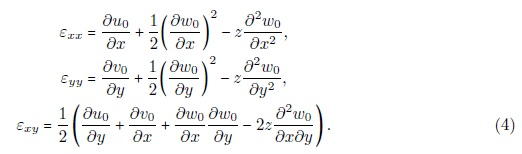We assume that the plate is made of linear elastic material and that the plane stress exists. Then the plane stress-reduced elastic constitutive equations are given bywhere the components of the elasticity matrix [Q] are given byHere E1 and E2 denote the elastic moduli along the principal material coordinate directions, which are assumed to coincide with the plate x and y-directions, ν12 and ν21 are Poisson's ratios, and G12 is the shear modulus.

By using the constitutive relations given in Eq. (5) and the definitions of the resultants given in the (2), we obtain the following plate constitutive relations: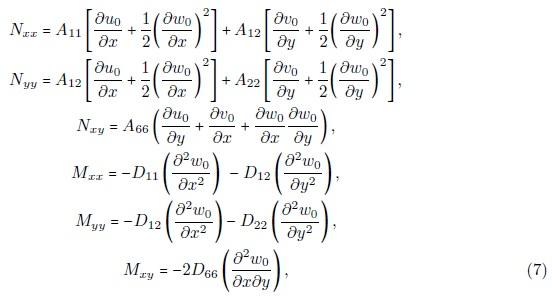where the plate extensional and bending stiffnesses are defined asfor i, j= 1, 2, 6 .

2.2 The First Order Shear Deformation Theory

The displacement field of the FSDT is given byThe von Karman nonlinear strains of the FSDT are given byThe plate constitutive equations in the FSDT are given bywhere, Ks (=5/6) is the shear correction factor. We introduce the effective shear forces as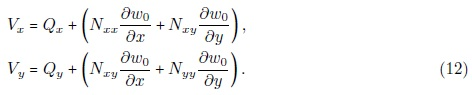3 FINITE ELEMENT MODELS

3.1 Summary of equations

In this section, we develop various types of the nonlinear mixed finite element models of plates. In current models, various stress resultants are included as independent nodal variables with the weighted-residual statements of suitable equations. Two new CPT models and two new FSDT models are developed. Keeping the forthcoming developments in mind the governing equations of the two theories are summarized first.

Governing equations of the CPTandGoverning equations of the FSDTandwhereandare inverses of the stiffness matrices: A* =A-1 and D* =D-1 .

3.2 Finite Element Model I (CPT)

In this mixed finite model of the CPT, eleven variables, u0, v0, w0, Nxx, Nyy, Nxy, Vx, Vy, Mxx, Myy and Mxy, are treated as independent variables. The following weighed-residual statements are used: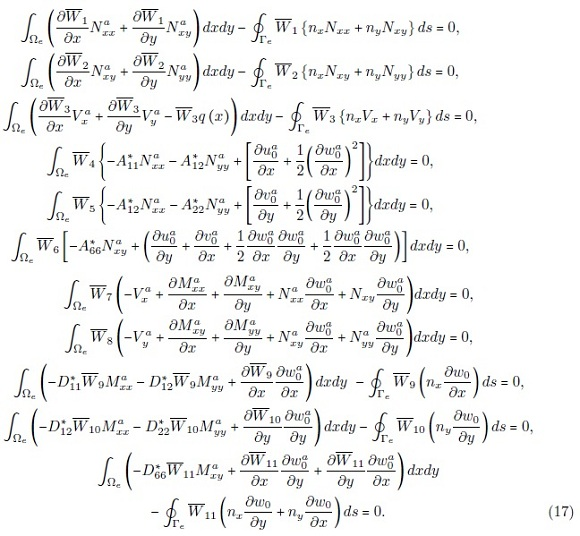where, Γe is the boundary of a typical element region Ωe, variables with a superscript 'a' denote the approximated variables, and nx and ny denote the x and y components (i.e. direction cosines) of the unit normal vector on the boundary. The primary variables and the secondary variable of the formulation are as follows.With the weighted residual statements in (17), we can develop the finite element model, denoted as Model I, of the CPT by approximating the 11 variables with known interpolation functions and unknown nodal values. The Lagrange interpolation functions are admissible for all variables (i.e., C0 continuity of all variables is required). We takeBy substituting the expressions from (18) into the weighted-residual statements of (17), we obtain the finite element equationswhereThe rest of the coeffcients matrices and force vectors are zero.

3.3 Finite Element Model II (CPT)

The shear forces Vx and Vy can be eliminated by substituting the forth and the fifth equilibrium equations into the third equilibrium equation of the CPT. The following 9 weighted-residual statements are used:All 9 variables are approximated with the Lagrange type interpolation functions, and the finite element model is of the form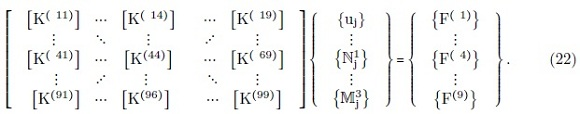where the coeffcients can be easily identified from the weighted-residual statements in (20).

3.4 Finite Element Model III (FSDT)

Model III is based on the following 13 weighed-residual statements of the FSDT: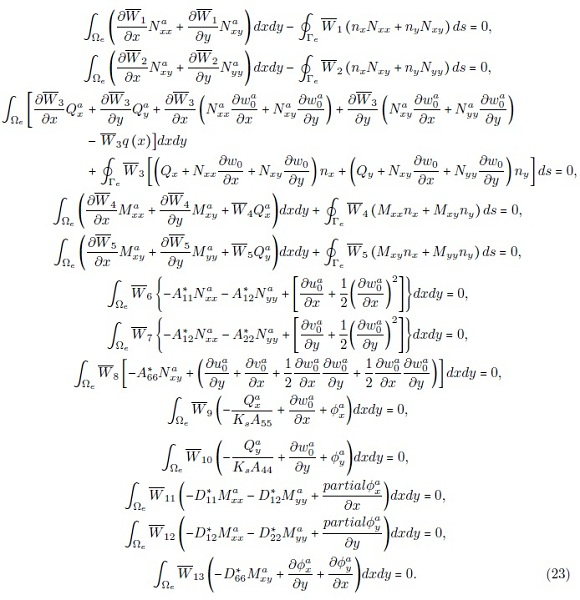where Ωe and Γe denote the element region and its boundary, respectively. The primary variables and the secondary variable of the Model III can be specified as follows:The finite element model is of the form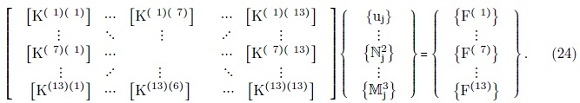The coeffcients can be identified with the help of the weighted-residual statements in (23).

3.5 Finite Element Model IV (FSDT)

The in-plane forces (Nxx, Nxy and Nyy) can be eliminated by substituting from the first two equilibrium equations into the remaining equations of equilibrium. The weighted-residual statements of the resulting 10 equations are summarized below: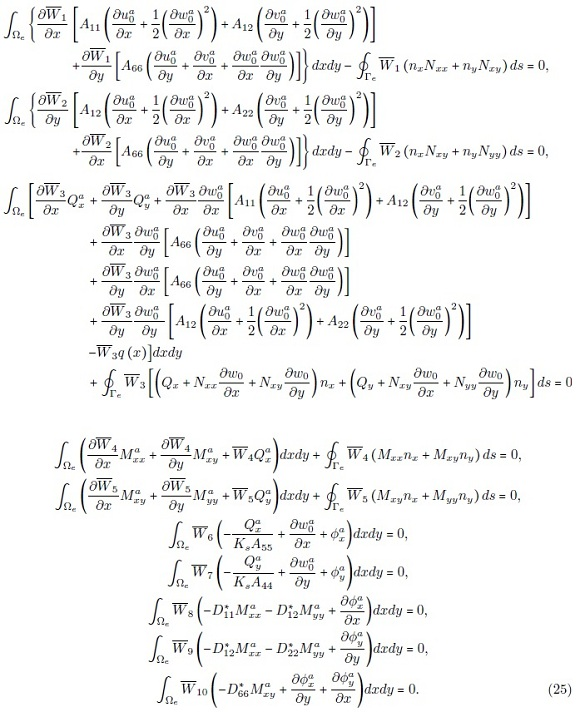The primary and the secondary variables of Model IV are the same as in Model III. The finite element model is of the form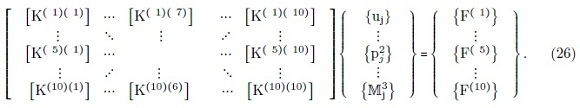where the nonzero coeffcients are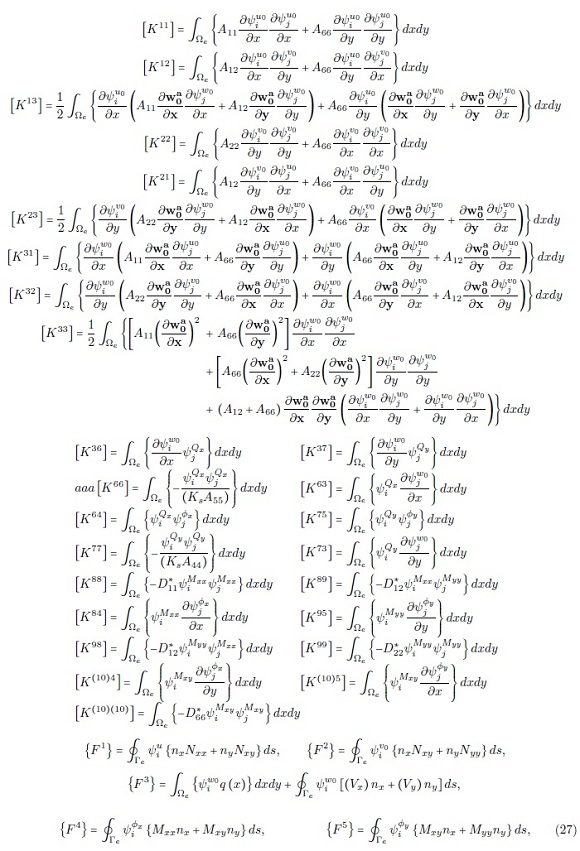4 NUMERICAL RESULTS

In this section we will discuss the numerical results obtained with the finite element models developed in Section 3. Comparisons of various models are presented with linear and nonlinear solutions available in the literature. The Newton's iterative technique is used to solve the nonlinear equations. The tangent stiffness coeffcients are computed from the stiffness coeffcients (see Reddy  for details).

We consider a square plate with the following material properties: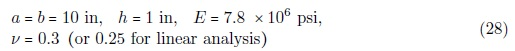Due to the biaxial symmetry of the geometry, boundary conditions, and applied load, only a quadrant of the plate was used as the computational domain. Three types of boundary conditions are considered with common boundary conditions along the symmetry lines of the quadrant. The specific boundary conditions are shown in Fig. 2.

4.1 Linear analysis

To verify the accuracy of the newly developed plate bending models, solutions obtained with the new models are compared with those of the existing models [1, 2, 6] and analytic solutions. First, the linear solutions of the mixed CPT models will be discussed by comparing the results obtained with displacement based model [4, 6].

The comparison of the results of the various models under the simple support I (SS1) and clamped (CC) boundary conditions are given in Tables 1 and 2. For the simple support boundary condition (SS1), Model II showed best accuracy for the center vertical deflection, while Model I provided better accuracy for the center bending moment, as shown in Table 1. For the clamped (CC) boundary condition, the Model I showed best accuracy both for the center vertical deflection and the center bending moment as shown in Table 2. By including the shear forces (i.e., Vx and Vy) as nodal values in Model I, more accurate center bending moment and center vertical deflection were obtained.

Current CPT mixed models were compared with the displacement based model. For the CPT displacement based model, non-conforming and the conforming  elements should be used because of the continuity requirement of the weak formulation. Current mixed models provided better accuracy when the compatible nine-node quadratic element was used. Even the four-node liner element also provided acceptable accuracy compared with the non-conforming displacement based model. The stresses obtained from the current mixed models showed better accuracy, because the stresses can be directly computed by using bending moment or shear resultant obtained at a node.

Next, the numerical results of the Model III and IV are compared with the results of Reddy's mixed model  in Table 3. The mixed model developed by Reddy  included bending moments as independent nodal value in the finite element model, while current Model III and IV included vertical shear resultants (i.e., Qx and Qy), as independent nodal value. Note that the difference between Model III and VI comes from the presence or absence of membrane forces (i.e., Nxx, Nyy and Nxy) in the finite element models. Thus, the solution of the linear bending of each model is essentially the same as shown in Table 3.

4.2 Nonlinear analysis

A total of 12 load steps were used with the following values of the load parameter P = q0a4/ (E22h4):

P = { 6.25, 12.5, 25.0, 25.0, 25.0, 25.0, 25.0, 25.0, 25.0, 25.0, 25.0, 25.0 } (29)

A tolerance ϵ = 0.01 was used for convergence in the Newton's iteration scheme. Model I and II was compared with the CPT displacement base model to see its non-linear behavior. The center defection, w0, of the newly developed models are presented in Table 4. In every load step, the converged solution was obtained within 4 iterations. Results of full integration and the reduced integration are presented in Table 4. In both models both membrane and shear locking are not severe, as judged against the published solutions, and the effect of reduced integration is not significant.

The nonlinear load vs. deflection and load vs. stress are presented in Fig. 3. For the SS3 boundary condition, both vertical deflection and stresses of Models I and II showed very close agreement with the displacement finite element model. The normal stresses and the membrane stresses were computed at points (0, 0, 0.5h) and (0, 0, 0), respectively. The 9-node quadratic element mesh showed closer agreement with the displacement FSDT model.

The nonlinear center deflection, normal and membrane stresses of Models I and II are compared with the results of the displacement model. The results are presented in Table 5. A4×4 mesh of nine-node element showed the closest agreement with the displacement FSDT model, as shown in Fig. 4. To see the convergence of the various models, center deflections of previously developed models with 2×2 quadratic and 4×4 linear meshes under SS1 and SS3 boundary conditions are compared in Table 6. Every model showed good convergence with a tolerance ϵ =0.01, except for the Model IV. The Model IV showed acceptable convergence with SS3 boundary condition but with SS1 boundary condition it took slightly more iterations to converge. This is due to the fact that plates with SS1 boundary conditions are more flexible and exhibit greater nonlinearity.

The distributions of various quantities are presented in Figs. 5 and 6. The data was post-processed inside of each element using 10 Gauss points ranging from -0.975 to 0.975, for both newly developed models (i.e., Models I and III) and FSDT displacement model. Converged solutions of SS3 at load parameter P = 250.0 are used for the post processing.

Even though all models show similar patterns for each variable as shown in Fig. 5, one may note that the contour plots obtained from the current mixed models offer better accuracy at the boundaries of the elements, while the plots obtained from the displacement based model show discontinuous distributions. Obviously, the plots in Figs. 6 and 4.6 show that the distributions of stresses and bending moments of Model III are relatively more accurate than those computed in the displacement model of FSDT, even though bending moments of Model III have some oscillations at the inter-element boundary. Of course, the displacement models exhibit even higher discontinuities in the bending moments as well as shear forces.

5 CONCLUSIONS

In this study, advantages and disadvantages of newly developed nonlinear finite element models of plate bending are investigated. In almost every case, newly developed mixed plate bending models provided better accuracy for linear and nonlinear solutions of deflections and stress resultants. Model IV showed poor convergence compared with other models because of the absence of typical displacement variables. An important observation of the present study is that the mixed models do not experience significant locking.

In summary, the two main advantages of the mixed model are the reduction in the continuity requirements for the transverse deflection in CPT and the increase of the accuracy for the stress resultants. Of course, there is a slight increase in computational cost due to the increased number of degrees of freedom per node.

Acknowledgement. The second author gratefully acknowledges the support of this research by Army Research Offce.

Received 2 Feb 2010; In revised form 15 Mar 2010

•  D. J. Allman. Triangular finite element for plate bending with constant and linearly varying bending moments. High Speed Computing of Elastic Structures I, pages 106-136, 1971.
•  L. R. Herrmann. Finite element bending analysis for plates. J. Engng. Mech. Div. , ASCE, 93(EM5), 1967.
•  N. S. Putcha and J. N. Reddy. A refined mixed shear flexible finite element for the nonlinear analysis of laminated plates. Computers & Structures, 22(4):529-538, 1986.
•  J. N. Reddy. Mixed finite element models for laminated composite plate. Journal of Engineering for Industry, 109:39-45, 1987.
•  J. N. Reddy. Energy Principles and Variational Methods in Applied Mechanics. John Wiley & Sons, New York, 2 edition, 2002.
•  J. N. Reddy. An Introduction to Nonlinear Finite Element Analysis. Oxford University Press, Oxford, New York, 2004.
•  J. N. Reddy. Mechanics of Laminated Composite Plates and Shells : Theory and Analysis. CRC Press, Boca Raton, 2 edition, 2004.
•  J. N. Reddy. An Introduction to the Finite Element Method. McGraw-Hill, New York, 3 edition, 2006.
•  J. N. Reddy. Theory and Analysis of Elastic Plates and Shells. CRC Press, Boca Raton, FL, 2007.
•  J. N. Reddy. An Introduction to Continuum Mechanics with Applications. Cambridge University Press, New York, 2008.
• *
Author email:
+
currently at Department of Mechanical Engineering, Korea Army Academy at Yeong cheon, Yeong cheon, 770-849 South Korea

# Publication Dates

• Publication in this collection
12 Mar 2012
• Date of issue
2010